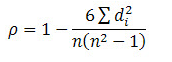# Sandeep Garg Solutions Class 11 Economics Chapter 8 - Measures of Correlation

Sandeep Garg Class 11 Economics Solutions Economics Chapter 8 – Measures of Correlation are illustrated by the professional economic educator from the contemporary edition of Sandeep Garg Economics Class 11 textbook solutions.

We at BYJU’S provide Sandeep Garg Economics Class 11 Solutions to give a comprehensive insight into the subject to the students. These insights will be a valuable advantage to students while completing their homework or while studying for their exams.

Here are some questions and their solutions on the tabular representation.

## Sandeep Garg Solutions Class 11 – Chapter 8

Question1

Evaluate the coefficient of rank correlation of ten artisans of a facility that is ranked in terms of their capability by 2 separate judges:

 Artisans Name P Q R S T U V W X Y Position given by Judge P 2 9 5 5 2 2 4 7 8 10 Position given by Judge R 3 8 5 7 2 3 2 5 10 9

 Artisans Name Position given by Judge RP Position given by Judge RR D= RP−RR D2 P Q R S T U V W X Y 2 9 5 5 2 2 4 7 8 10 3 8 5 7 2 3 2 5 10 9 -1 1 0 -2 0 -1 2 2 -2 1 1 1 0 4 0 1 4 4 4 1 ΣD2=20

N= 10Or, ρ = 1-

$$\begin{array}{l}\frac{6X20}{1000-10}\end{array}$$

ρ= 1 – 0.121

ρ = 0.88

Hence, the coefficient of rank correlation is 0.88

Question 2

The following are marks of 8 students in Maths and Accountancy. Evaluate the coefficient of rank correlation.

 Maths Marks 50 60 65 70 75 40 80 85 Accountancy Marks 80 71 60 75 90 82 70 50

 Maths Marks Accountancy Marks R1 R2 D= R1−R2 D2 50 60 65 70 75 40 80 85 80 71 60 75 90 82 70 50 2 3 4 5 6 1 7 8 6 4 2 5 8 7 3 1 −4 −1 2 0 −2 −6 4 7 16 1 4 0 4 36 16 49 ΣD2=126

N= 8Or, ρ = 1-

$$\begin{array}{l}\frac{6X126}{512-8}\end{array}$$

ρ = 1- 1.5

ρ = 0.5

Hence, the coefficient of rank correlation is 0.5

Question 3

What is the coefficient of rank correlation of the following information

 Subject A 3 5 8 4 7 10 2 1 6 9 Subject B 6 4 9 8 1 2 3 10 5 7

 RA RB D = RA− RB D2 3 5 8 4 7 10 2 1 6 9 6 4 9 8 1 2 3 10 5 7 −3 1 −1 −4 6 8 −1 −9 1 2 9 1 1 16 36 64 1 81 1 4 ΣD2=214

N= 10ρ = 1 –

$$\begin{array}{l}\frac{6\sum D^{2}}{N^{3}-N}\end{array}$$

Or, ρ = 1-

$$\begin{array}{l}\frac{6X214}{1000-10}\end{array}$$

ρ = 1- 1.2969

Hence, the coefficient of rank correlation is -0.2969

Question 4

Evaluate rank correlation between advertisement sales and cost according to the following information

 Cost 78 36 98 25 75 82 90 62 65 39 Sales (in lakh ) 84 51 91 60 68 62 86 58 53 47

 Advertising Cost (X) Sales (Y) R1 R2 D= R1−R2 D2 78 36 98 25 75 82 90 62 65 39 84 51 91 60 68 62 86 58 53 47 7 2 10 1 6 8 9 4 5 3 8 2 10 5 7 6 9 4 3 1 −1 0 0 −4 −1 2 0 0 2 2 1 0 0 16 1 4 0 0 4 4 ΣD2=30

N= 10ρ = 1 –

$$\begin{array}{l}\frac{6\sum D^{2}}{N^{3}-N}\end{array}$$

Or, ρ = 1-

$$\begin{array}{l}\frac{6X30}{1000-10}\end{array}$$

ρ = 1 – 0.1818

=  0.818

Hence, the coefficient of rank correlation is 0.82

Question 5

Evaluate rank coefficient of correlation.

 X 1 2 3 4 5 6 7 8 9 10 11 12 Y 12 9 6 10 3 5 4 7 8 2 11 1

 X Y R1 R2 D=R1−R2 D2 1 2 3 4 5 6 7 8 9 10 11 12 12 9 6 10 3 5 4 7 8 2 11 1 1 2 3 4 5 6 7 8 9 10 11 12 12 9 6 10 3 5 4 7 8 2 11 1 −11 −7 −3 −6 2 1 3 1 1 8 0 11 121 49 9 36 4 1 9 1 1 64 0 121 N = 12 ΣD2=416

Here, m= 0

N= 12ρ = 1 –

$$\begin{array}{l}\frac{6\sum D^{2}}{N^{3}-N}\end{array}$$

ρ = 1-

$$\begin{array}{l}\frac{6X416}{1728-12}\end{array}$$

Or, ρ = 1 –

$$\begin{array}{l}\frac{2496}{1716}\end{array}$$

ρ = 1- 1.4545

= -0.4545

Hence, the coefficient of rank correlation is – 0.4545

The above-provided solutions are considered to be the best solution for ‘Sandeep Garg Economics Class 11 Solutions Chapter 8 – Measures of Correlation. Stay tuned to BYJU’S to learn more.